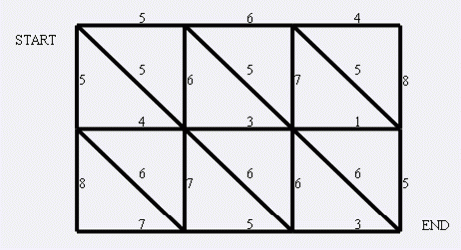# bzoj1001/洛谷 P4001 [BJOI2006]狼抓兔子 题解【网络流】【最小割】

弃isap从dinic之路……

## 题目描述1:(x,y)<==>(x+1,y)

2:(x,y)<==>(x,y+1)

3:(x,y)<==>(x+1,y+1)

## 输入输出样例

3 4
5 6 4
4 3 1
7 5 3
5 6 7 8
8 7 6 5
5 5 5
6 6 6

14

## 题解：

isap太难调了……过不了样例以为是isap问题，结果改写dinic发现还是过不了？但是写了写dinic发现dinic好写太多了常数还小qaq。

这个题题意要求就是最小割，但是这个题是可以反向跑的，也就是说路是双向的。我们是否需要建立双向的流边呢？

我们知道，增广一条道路时，会把它的反向边增加上正向边减小的值。这样也相当于成环，而我们如果独立地建立双向流边，达成的效果是一样的，因此可以在一开始加边的时候，就把反向流量设为同样的大小，这样既满足题意，又符合我们的思维，也方便了增广路的运作。（如果建立两组边的话，处理不好会MLE）

### 吐槽isap:

isap要开的数组太多了。。。还有三四个细节每次写都会出错……就当学会了一种思维吧。还是dinic大法好（逃

## Code：

#include<cstdio>
#include<cstring>
struct edge
{
int n,v;
int nxt;
edge(int n,int nxt,int v)
{
this->n=n;
this->nxt=nxt;
this->v=v;
}
edge(){}
}e;
{
}
int n,m;
int pla(int x,int y)
{
return (x-1)*m+y;
}
int sum=0;
int q,l=0,r=0;
int d,cur;
bool bfs()//bfs过程
{
memset(d,0,sizeof(d));
d=1;
l=0,r=0;
q[++r]=1;
while(l<r)
{
int x=q[++l];
if(e[i].v&&!d[e[i].n])
{
d[e[i].n]=d[x]+1;
q[++r]=e[i].n;
}
}
return d[n*m]>0;
}
int dinic(int x,int mx)
{
if(x==n*m)//到达终点
return mx;
int rst=mx;
for(int i=cur[x];(~i)&&rst;i=e[i].nxt)
if(e[i].v&&d[e[i].n]==d[x]+1)
{
int t=dinic(e[i].n,rst<e[i].v?rst:e[i].v);
if(!t)
d[e[i].n]=0;
e[i].v-=t;//增广
e[i^1].v+=t;
rst-=t;
}
return mx-rst;
}
int main()
{
int u,v,w;
scanf("%d%d",&n,&m);
for(int i=1;i<=n;++i)
for(int j=1;j<m;++j)
{
scanf("%d",&w);
}
for(int i=1;i<n;++i)
for(int j=1;j<=m;++j)
{
scanf("%d",&w);
}
for(int i=1;i<n;++i)
for(int j=1;j<m;++j)
{
scanf("%d",&w);
}
int sum=0;
while(bfs())
{
int t=dinic(1,0x3fffffff);
while(t)
{
sum+=t;
t=dinic(1,0x3fffffff);
}
}
printf("%d\n",sum);
return 0;
}


### 5 说点什么0 Followers

Most reacted comment
0 Comment authors
Recent comment authors
Subscribe[…] Read More to that Topic: wjyyy.top/1540.html […][…] Information on that Topic: wjyyy.top/1540.html […]wpDiscuz